International
Tables for
Crystallography
Volume D
Physical properties of crystals
Edited by A. Authier

International Tables for Crystallography (2006). Vol. D, ch. 2.2, pp. 295-296

## Section 2.2.4. The Bloch theorem

K. Schwarza*

aInstitut für Materialchemie, Technische Universität Wien, Getreidemarkt 9/165-TC, A-1060 Vienna, Austria
Correspondence e-mail: kschwarz@theochem.tuwein.ac.at

### 2.2.4. The Bloch theorem

| top | pdf |

The electronic structure of an infinite solid looks so complicated that it would seem impossible to calculate it. Two important steps make the problem feasible. One is the single-particle approach, in which each electron moves in an average potentialaccording to a Schrödinger equation,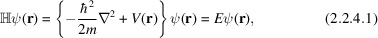and has its kinetic energy represented by the first operator. The second important concept is the translational symmetry, which leads to Bloch functions. The single-particle aspect will be discussed later (for details see Sections 2.2.9and 2.2.10).

#### 2.2.4.1. A simple quantum-mechanical derivation

| top | pdf |

In order to derive the Bloch theorem, we can simplify the problem by considering a one-dimensional case with a lattice constant a. [The generalization to the three-dimensional case can be done easily according to (2.2.3.15).] The one-dimensional Schrödinger equation is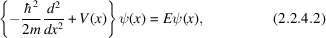where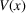is invariant under translations, i.e.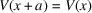. We define a translation operator t according to (2.2.3.1)for the translation by one lattice constant as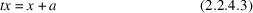and apply its functional counterpartto the potential, which gives [according to (2.2.3.4)]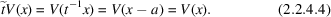The first part in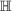corresponds to the kinetic energy operator, which is also invariant under translations. Therefore, since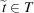(the lattice translation subgroup) and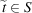(the Schrödinger group),commutes with, i.e. the commutator vanishes,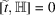or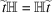. This situation was described above [see (2.2.3.16)–(2.2.3.18)] and leads to the fundamental theorem of quantum mechanics which states that when two operators commute the eigenvectors of the first must also be eigenvectors of the second. Consequently we have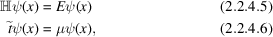whereis the eigenvalue corresponding to the translation by the lattice constant a. The second equation can be written explicitly as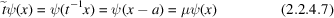and tells us how the wavefunction changes from one unit cell to the neighbouring unit cell. Notice that the electron density must be translationally invariant and thus it follows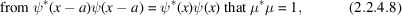which is a necessary (but not sufficient) condition for defining.

#### 2.2.4.2. Periodic boundary conditions

| top | pdf |

We can expect the bulk properties of a crystal to be insensitive to the surface and also to the boundary conditions imposed, which we therefore may choose to be of the most convenient form. Symmetry operations are covering transformations and thus we have an infinite number of translations in T, which is most inconvenient. A way of avoiding this is provided by periodic boundary conditions (Born–von Karman). In the present one-dimensional case this means that the wavefunction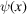becomes periodic in a domain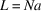(with integer N number of lattice constants a), i.e.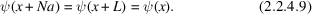According to our operator notation (2.2.4.6), we have the following situation when the translation t is applied n times: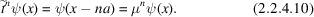It follows immediately from the periodic boundary condition (2.2.4.9)that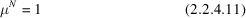with the obvious solution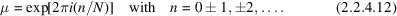Here it is convenient to introduce a notation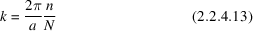so that we can write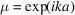. Note that k is quantized due to the periodic boundary conditions according to (2.2.4.13). Summarizing, we have the Bloch condition (for the one-dimensional case):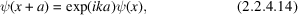i.e. when we change x by one lattice constant a the wavefunction at x is multiplied by a phase factor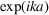. At the moment (2.2.4.13)suggests the use of k as label for the wavefunction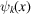.

Generalization to three dimensions leads to the exponential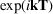with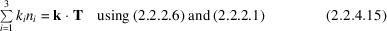and thus to the Bloch condition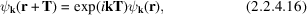or written in terms of the translational operator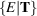[see (2.2.3.15)]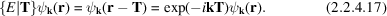The eigenfunctions that satisfy (2.2.4.17)are called Bloch functions and have the form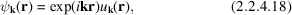where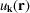is a periodic function in the lattice,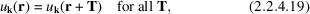andis a vector in the reciprocal lattice [see (2.2.2.6)] that plays the role of the quantum number in solids. Thevector can be chosen in the first BZ, because any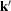that differs fromby just a lattice vectorof the reciprocal lattice has the same Bloch factor and the corresponding wavefunction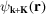satisfies the Bloch condition again, since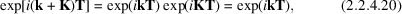where the factor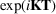is unity according to (2.2.2.7). Since these two functions,and, belong to the same Bloch factorthey are equivalent. A physical interpretation of the Bloch states will be given in Section 2.2.8.

#### 2.2.4.3. A simple group-theoretical approach

| top | pdf |

Let us repeat a few fundamental definitions of group theory: For any symmetry operation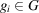, the product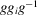can always be formed for anyand defines the conjugate element ofby g. Given any operation, its class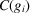is defined as the set of all its conjugates under all operations. What we need here is an important property of classes, namely that no two classes have any element in common so that any group can be considered as a sum of classes.

Assuming periodic boundary conditions with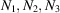number of primitive cells along the axes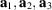, respectively, a lump of crystal with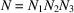unit cells is studied. The translation subgroup T contains the general translation operators, which [using (2.2.3.15)] can be written as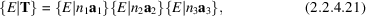where each factor belongs to one of the three axes. Since T is commutative (Abelian), each operation of T is its own class and thus the number of classes equals its order, namely N. From the general theorem that the squares of the dimensions of all irreducible representations of a group must equal the order of the group, it follows immediately that all N irreducible representations of T must be one-dimensional (see also Section 1.2.3.2of the present volume). Taking the subgroup along theaxis, we must havedifferent irreducible representations, which we label (for later convenience) byand denote as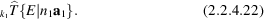These representations are one-dimensional matrices, i.e. numbers, and must be exponentials, often chosen of the form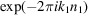. The constantmust be related to the corresponding label of the irreducible representation. In the three-dimensional case, we have the corresponding representation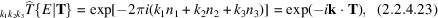where we have used the definitions (2.2.2.6)and (2.2.2.1). Within the present derivation, the vectorcorresponds to the label of the irreducible representation of the lattice translation subgroup.